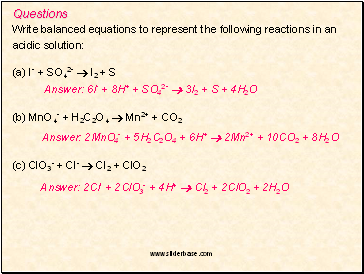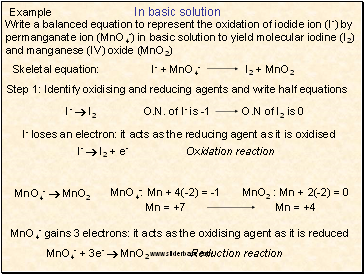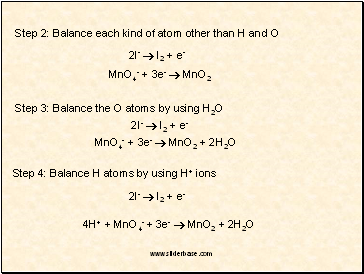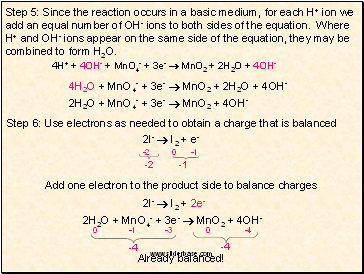# Balancing Chemical Equations. Balancing redox equationsPage 6

#### WATCH ALL SLIDES

8H+ + MnO4- + 5e-  Mn2+ + 4H2O

× 2

2H2O + SO2  SO42- + 2e- + 4H+

× 5

16H+ + 2MnO4- + 10e-  2Mn2+ + 8H2O

10H2O + 5SO2  5SO42- + 10e- + 20H+

16H+ + 2MnO4- + 10H2O + 5SO2  2Mn2+ + 8H2O + 5SO42- + 20H+

2MnO4- + 2H2O + 5SO2  2Mn2+ + 5SO42- + 4H+

0

0

-2

-2

+4

Balanced

Check to ensure that all atoms and charges are balanced.

Slide 24Questions

Write balanced equations to represent the following reactions in an

acidic solution:

(a) I- + SO42-  I2 + S

Answer: 6I- + 8H+ + SO42-  3I2 + S + 4H2O

(b) MnO4- + H2C2O4  Mn2+ + CO2

Answer: 2MnO4- + 5H2C2O4 + 6H+  2Mn2+ + 10CO2 + 8H2O

(c) ClO3- + Cl-  Cl2 + ClO2

Answer: 2Cl- + 2ClO3- + 4H+  Cl2 + 2ClO2 + 2H2O

Slide 25In basic solution

Example

Write a balanced equation to represent the oxidation of iodide ion (I-) by permanganate ion (MnO4-) in basic solution to yield molecular iodine (I2) and manganese (IV) oxide (MnO2)

Step 1: Identify oxidising and reducing agents and write half equations

I-  I2

O.N. of I- is -1

O.N of I2 is 0

I- loses an electron: it acts as the reducing agent as it is oxidised

I-  I2 + e- Oxidation reaction

MnO4-  MnO2

MnO4-: Mn + 4(-2) = -1

Mn = +7

MnO2 : Mn + 2(-2) = 0

Mn = +4

MnO4- gains 3 electrons: it acts as the oxidising agent as it is reduced

MnO4- + 3e-  MnO2 Reduction reaction

Skeletal equation: I- + MnO4- I2 + MnO2

Slide 26Step 2: Balance each kind of atom other than H and O

2I-  I2 + e-

MnO4- + 3e-  MnO2

Step 3: Balance the O atoms by using H2O

2I-  I2 + e-

MnO4- + 3e-  MnO2 + 2H2O

Step 4: Balance H atoms by using H+ ions

2I-  I2 + e-

4H+ + MnO4- + 3e-  MnO2 + 2H2O

Slide 27Step 5: Since the reaction occurs in a basic medium, for each H+ ion we add an equal number of OH- ions to both sides of the equation. Where H+ and OH- ions appear on the same side of the equation, they may be combined to form H2O.

4H+ + 4OH- + MnO4- + 3e-  MnO2 + 2H2O + 4OH-

4H2O + MnO4- + 3e-  MnO2 + 2H2O + 4OH-

2H2O + MnO4- + 3e-  MnO2 + 4OH-

Step 6: Use electrons as needed to obtain a charge that is balanced

2I-  I2 + e-

-2

-1

Add one electron to the product side to balance charges

2I-  I2 + 2e-

2H2O + MnO4- + 3e-  MnO2 + 4OH-

-4

-4